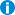# Νέα / Ανακοινώσεις / Συζητήσεις

• Όλες οι κατηγορίες
• Δημόσιες Ανακοινώσεις
• Διαλέξεις / Ημερίδες / Εκδηλώσεις
• Διάλεξη του Prof. Matania Ben-Artzi, Einstein Institute of Mathematics: "High-order simulation of N-S equations in 2-D". 18-4-2019, ώρα 12.00, Αίθουσα Γ2.2

# Διάλεξη του Prof. Matania Ben-Artzi, Einstein Institute of Mathematics: "High-order simulation of N-S equations in 2-D". 18-4-2019, ώρα 12.00, Αίθουσα Γ2.2

• Συντάχθηκε 16-04-2019 18:38Ενημερώθηκε: -

Ο Prof. Matania Ben-Artzi, Professor of Mathematics στο Einstein Institute of Mathematics, Hebrew University of Jerusalem, θα δώσει διάλεξη την Πέμπτη 18-4-2019 και ώρα 12.00 (Αμφιθέατρο Γ2.2, δίπλα στη Σχολή Μ.Π.Δ.) με τίτλο: "High-order simulation of Navier-Stokes equations in 2-D".

Ακολουθεί περίληψη της διάλεξης:

A pure-streamfunction formulation is introduced for the theoretical study and numerical simulation of the 2-D incompressible Navier-Stokes equations. On the theoretical level, it leads to a nonlinear evolution equation involving the bi-laplacian. The basic mathematical results are concerned with the well-posedness of the system subject to "rough" initial data (such as point vortices). For the numerical aspect  of the talk , a new compact scheme is presented, which allows for a pure streamfunction formulation, very close to the theoretical treatment. This scheme is robust and, by using only the streamfunction, does not require vorticity boundary conditions (a major difficulty of vortex methods).

The scheme is analyzed for stability and convergence. It is shown to converge for the full nonlinear system. Numerical experiments are presented, including driven and double-driven cavities. For sufficiently high Reynolds numbers, the solution bifurcates, leading to symmetry breaking patterns and periodic solutions. (Joint work with J.-P. Croisille, D. Fishelov and S. Trachtenberg)."

### Συνημμένα:

• matania-cv.2018-short.pdf
Μεταφορτώσεις: 2042, Μέγεθος: 67 KB application/pdf